Quality and Process Methods > Measurement Systems Analysis > Statistical Details for Measurement Systems Analysis
Publication date: 05/24/2021

# Statistical Details for Measurement Systems Analysis

For more information about the calculations of the limits used in the Range Chart, see Control Limits for XBar and R Charts. For more information about the calculations of the limits used in the Standard Deviation Chart, see Control Limits for XBar and S Charts.

## Computation of Intraclass Correlation and Probable Error

Intraclass Correlation without bias is computed as follows: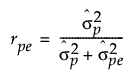Intraclass Correlation with bias is computed as follows: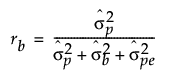Intraclass Correlation with bias and interaction factors is computed as follows: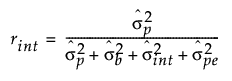Probable Error is computed as follows: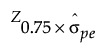Note the following: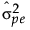= variance estimate for pure error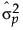= variance estimate for product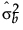= variance estimate for bias factors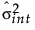= variance estimate for interaction factors

Z0.75 = the 75% quantile of standard normal distribution